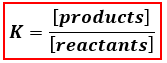# Problem: Write chemical equations and corresponding equilibrium expressions for each of the two ionization steps of carbonic acid.Express as chemical equation; identify all phases.a. Write chemical equations for first ionization step of carbonic acid.b. Write chemical equations for second ionization step of carbonic acid.

###### FREE Expert Solution

We are being asked to write the equilibrium expressions for each of the two ionization steps of carbonic acid.

Recall that equilibrium expressions are written as:Carbonic acid:###### Problem Details

Write chemical equations and corresponding equilibrium expressions for each of the two ionization steps of carbonic acid.

Express as chemical equation; identify all phases.

a. Write chemical equations for first ionization step of carbonic acid.

b. Write chemical equations for second ionization step of carbonic acid.